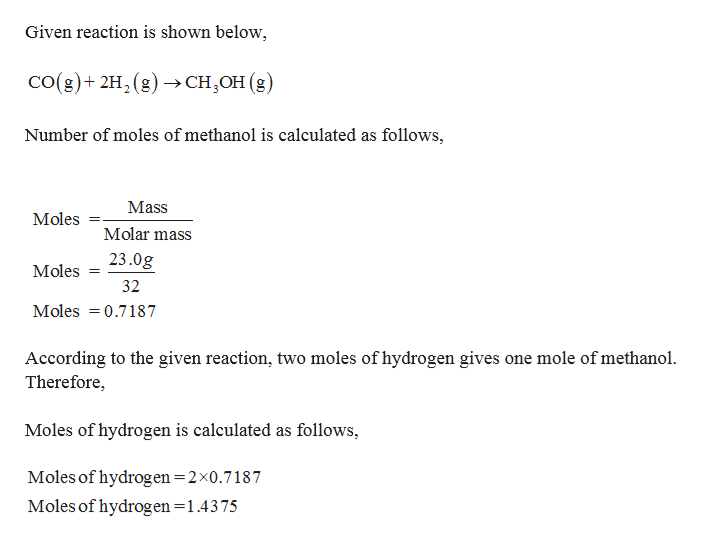# CH3OH can be synthesized by the following reaction. CO(g)+2H2(g)→CH3OH(g)What volume of H2 gas (in L), measured at 758 mmHg and 90 ∘C, is required to synthesize 23.0 g CH3OH?

Question
14 views

CH3OH can be synthesized by the following reaction.
CO(g)+2H2(g)→CH3OH(g)

What volume of H2 gas (in L), measured at 758 mmHg and 90 ∘C, is required to synthesize 23.0 g CH3OH?

check_circle

Step 1

Given,

Methanol is 23.0 g

T = 273 + 90 = 363 K.

Pressure = 758 m...help_outlineImage TranscriptioncloseGiven reaction is shown below co(g)+ 2H2 (g)CH,OH (g) Number of moles of methanol is calculated as follows, Mass Moles Molar mass 23.0g Moles 32 Moles 0.7187 According to the given reaction, two moles of hydrogen gives one mole of methanol. Therefore, Moles of hydrogen is calculated as follows Moles of hydrogen =2x0.7187 Moles of hydrogen -1.4375 fullscreen

### Want to see the full answer?

See Solution

#### Want to see this answer and more?

Solutions are written by subject experts who are available 24/7. Questions are typically answered within 1 hour.*

See Solution
*Response times may vary by subject and question.
Tagged in

### Chemistry